# Argument, principle of the

(diff) ← Older revision | Latest revision (diff) | Newer revision → (diff)

argument principle

A geometric principle in the theory of functions of a complex variable. It is formulated as follows: Letbe a bounded domain in the complex plane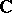, and let, moreover, the boundary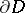be a continuous curve, oriented so thatlies on the left. If a function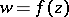is meromorphic in a neighbourhood ofand has no zeros or poles on, then the difference between the number of its zerosand the number of its poles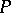inside(counted according to their multiplicity) is equal to the increase of the argument ofwhen travelling once around, divided by, i.e.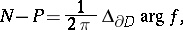where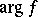denotes any continuous branch ofon the curve. The expression on the right-hand side equals the index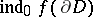of the curve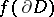with respect to the point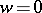.

The principle of the argument is used in the proofs of various statements on the zeros of holomorphic functions (such as the fundamental theorem of algebra on polynomials, the theorem of Hurwitz on zeros, etc.). From the principle of the argument follow many other important geometric principles of function theory, e.g. the principle of invariance of domain (cf. Invariance, principle of), the maximum-modulus principle and the theorem on the local inverse of a holomorphic function. In many questions the principle of the argument is used implicitly, in the form of its corollary: the Rouché theorem.

There are generalizations of the principle of the argument. The condition thatbe meromorphic in a neighbourhood of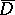may be replaced by the following:has only a finite number of poles and zeros inand extends continuously to. Instead of the complex plane, an arbitrary Riemann surface may be considered: the boundedness ofis then replaced by the condition thatbe compact. From the principle of the argument for a compact Riemann surface it follows that the number of zeros of an arbitrary meromorphic function, not identically equal to zero, is equal to the number of poles. The principle of the argument for domains inis equivalent to the theorem on the sum of the logarithmic residues (cf. Logarithmic residue). For this reason, the following statement is sometimes called the generalized principle of the argument. Ifis meromorphic in a neighbourhood of a domainwhich is bounded by a finite number of continuous curves and ifhas no zeros or poles on, then for any functionwhich is holomorphic in a neighbourhood ofthe equalityholds, where the first sum extends over all zeros and the second sum extends over all poles ofin. There is also a topological generalized principle of the argument: The principle of the argument is valid for any open mappingthat is locally finite-to-one and extends continuously to, while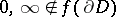.

An analogue of the principle of the argument for functions of several complex variables is, for example, the following theorem: Letbe a bounded domain in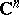with Jordan boundaryand letbe a holomorphic mapping of a neighbourhood ofsuch that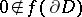; then the number of pre-images of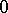in(counted according to multiplicity) is equal to.

How to Cite This Entry:
Argument, principle of the. Encyclopedia of Mathematics. URL: http://encyclopediaofmath.org/index.php?title=Argument,_principle_of_the&oldid=15915
This article was adapted from an original article by E.M. Chirka (originator), which appeared in Encyclopedia of Mathematics - ISBN 1402006098. See original article